# GSEB Solutions Class 10 Science Chapter 11 Human Eye and Colourful World

Gujarat Board GSEB Textbook Solutions Class 10 Science Chapter 11 Human Eye and Colourful World Textbook Questions and Answers, Additional Important Questions, Notes Pdf.

## Gujarat Board Textbook Solutions Class 10 Science Chapter 11 Human Eye and Colourful World

### Gujarat Board Class 10 Science Human Eye and Colourful World InText Questions and Answers

Question 1.
What is meant by the power of accommodation of the eye?
The ability of the eye to focus the distant objects as well as the nearby objects on the retina by changing the focal length of the eye lens is called the power of accommodation.

Question 2.
A person with a myopic eye cannot see objects beyond 1.2 m distinctly. What should be the type of the corrective lens used to restore proper vision?
A person with a myopic eye can use a concave lens to restore proper vision.Question 3.
What is the far point and near point of the human eye with normal vision?
The far point is infinity and the near point is 25 cm of the human eye with normal vision.

Question 4.
A student has difficulty reading the blackboard while sitting in the last row. What could be the defect the child is suffering from? How can it be corrected?
A student is suffering from the eye defect named myopia, in this defect person can see nearby objects clearly but cannot see far off objects distinctly. It can be corrected by using a concave lens.

In-Text Activities SolvedActivity 11.1

• Fix a sheet of white paper on a drawing board using drawing pins.
• Place a glass prism on it in such a way that it rests on its triangular base. Trace the outline of the prism using a pencil.
• Draw a straight line PE inclined to one of the refracting surfaces, say AB, of the prism.
• Fix two pins, say at points P and Q, on the line PE as shown in the figure below:
• Look for the images of the pins, fixed at P and Q, through the other face AC.
• two more pins, at points R and S, such that the pins at R and S and the images of the pins at P and Q lie on the same straight line.
• Remove the pins and the glass prism.
• The line PE meets the boundary of the prism at point E. Similarly, join and produce the points R and S. Let these lines meet the boundary of the prism at E and F, respectively. Join E and F.
• Draw perpendiculars to the refracting surfaces AB and AC of the prism at points E and F, respectively.
• Mark the angle of incidence (∠i), the angle of refraction (∠r) and the angle of emergence (∠e) as shown in the figure below.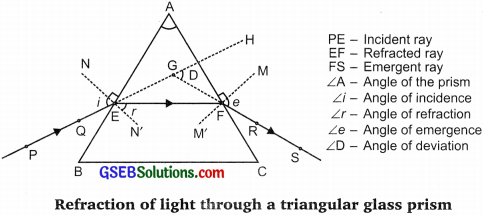Activity 11.2

• Take a thick sheet of cardboard and make a small hole or narrow slit in its middle.
• Allow sunlight to fall on the narrow slit. This gives a narrow beam of white light.
• Now take a glass prism and allow that light from the slit to fall on one of its faces as shown in the figure below.
• Turn the prism slowly until the light that comes out of it appears on a nearby screen.

Observation:
You will find a beautiful band of colours. This happens because prism split the white light that is incident on it and a band of colours is obtained. The blue light bends the most and red light bends the least.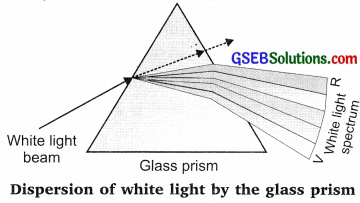Activity 11.3

• Place a strong source (S) of white light at the focus of a converging lens (L1). This lens provides a parallel beam of light.
• Allow the light beam to pass through a transparent glass tank (T) containing clear water.
• Allow the beam of light to pass through a circular hole (c) made in cardboard. Obtain a sharp image of the circular hole on a screen (MN) using a second converging lens (L2), as shown in the figure below.
• Dissolve about 200 g of sodium thiosulphate (hypo) in about 2 L of clean water taken in the tank. Add about 1 to 2 mL of concentrated sulphuric acid to the water. What do you observe?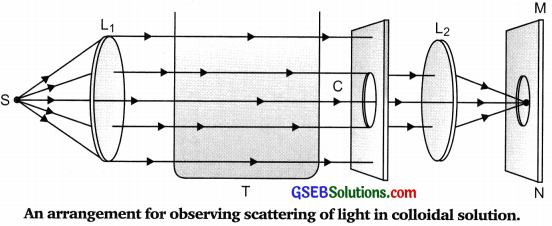Observation: The beaker appears to give blue light on its sides as the scattering of light takes place. While the light obtained on the screen is red in colour because all blue light has scattered and red light travels through the beaker, will not get scattered due to small particles in a beaker and passes straight and gets collected on the screen.

### Gujarat Board Class 10 Science Human Eye and Colourful World Textbook Questions and Answers

Question 1.
The human eye can focus objects at different distances by adjusting the focal length of the eye lens. This is due to
(a) presbyopia
(b) accommodation
(c) near-sightedness
(d) far-sightedness
(b) accommodation

Question 2.
The human eye forms the image of an object at its
(a) cornea
(b) iris
(c) pupil
(d) retina.
(d) retina.Question 3.
The least distance of distinct vision for a young adult with normal vision is about
(a) 25 m.
(b) 2.5 cm.
(c) 25 cm.
(d) 2.5 m.
(a) 25 cm

Question 4.
The change in focal length of an eye lens is caused by the action of the
(a) pupil
(b) retina
(c) ciliary muscles
(d) iris.
(C) ciliary muscles.

Question 5.
A person needs a lens of power 5.5 dioptres for correcting his distant vision. For correcting his near vision he needs a lens of power +1.5 dioptre. What is the focal length of the lens required for correcting (i) distant vision, and (ii) near vision?
The focal length of a lens is given by –
(p = $$\frac {1}{f}$$), f = $$\frac {1}{p}$$

(i) For distant vision
f = $$\frac {1}{-5.5D}$$ = -0.18 m

(ii) For near vision
f = $$\frac {1}{p}$$ i.e.,f = $$\frac {1}{1.5D}$$ = 0.67 m

Question 6.
The far point of a myopic person is 80 cm in front of the eye. What is the nature and power of the lens required to correct the problem?
For the myopic eye
u = – ∞
v = – 80 cm
f = ?
$$\frac {1}{v}$$ – $$\frac {1}{u}$$ = $$\frac {1}{f}$$
$$\frac {1}{-80cm}$$ – $$\frac {1}{-∞}$$ = $$\frac {1}{f}$$
f = -80 cm = – 0.80 m
power of the lens is
p = $$\frac {1}{f}$$
p = $$\frac {1}{-0.80 m}$$ = -1.25 D
A concave lens; p = -1.25 DQuestion 7.
Make a diagram to show how hypermetropia is corrected. The near point of a hypermetropic eye is 1 m. What is the power of the lens required to correct this defect? Assume that the near point of the normal eye is 25 cm.
u = -25 cm,
v = -1 m = -100 cm
$$\frac {1}{f}$$ = $$\frac {1}{v}$$ – $$\frac {1}{u}$$
= $$\frac {1}{-100}$$ – $$\frac {1}{(-25)}$$ = $$\frac {-1}{100}$$ + $$\frac {1}{25}$$
= $$\frac {1 + 4}{100}$$ = $$\frac {3}{100}$$ or f = $$\frac {1}{3}$$ m
p = $$\frac {1}{f}$$ = $$\frac {1}{1/3}$$ = 3D (convex lens)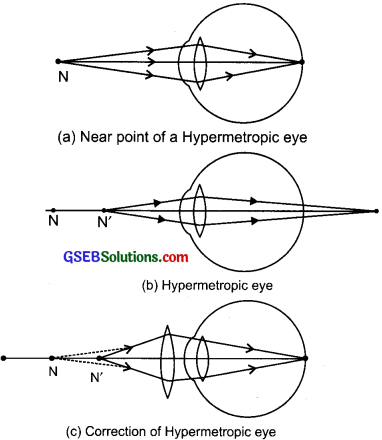Question 8.
Why is a normal eye not able to see clearly the objects placed closer than 25 cm?
Ciliary muscles can contract the Hypermetropic eye lens of the human eye to a certain limit because of which a person with normal vision can see the nearby objects clearly only if placed at 25 cm but if the object is placed closer to the eye than it cannot see the objects clearly.Question 9.
What happens to the image distance in the eye when we increase the distance of an object from the eye?
The image distance in the eye remains the same. On increasing the distance of an object from the eye, the focal length of the eye lens changes due to ciliary muscles which helps an eye to focus the object’s image on the retina.

Question 10.
Why do stars twinkle?
Stars twinkle due to atmospheric refraction of light from the stars and changing the density of the air around the earth.

Question 11.
Explain why the planets do not twinkle.
Planets are much closer to earth and are big enough in size.

Question 12.
Why does the sun appear reddish early in the morning?
When the sun rises early in the morning (or set in the evening), the light from the sun travels through the thicker layer of air and larger distance of the atmosphere surrounding the earth which makes the sunlight to scatter the most. Hence the blue light scatters the most but red light scatters the least and reaches our eyes.

Question 13.
Why does the sky appear dark instead of blue to an astronaut?
In space, there are no particles, air, gases, water droplets etc., present to scatter the light. So when the astronauts look at the sky in the space, there is no light entering our eyes, hence it appears dark.

### Gujarat Board Class 10 Science Human Eye and Colourful World Additional Important Questions and Answers

Question 1.
Name the transparent membrane through which light enters first in the eye.
Cornea.

Question 2.
What is the diameter of the human eye?
2.3 cm

Question 3.
Name the light-sensitive part of the eye where the image of an object is formed.
RetinaQuestion 4.
What is the function of iris?
Iris controls the size of the pupil.

Question 5.
What are light-sensitive cells?
Rods and cones.

Question 6.
What type of image is formed on the retina?
Real, inverted image.

Question 7.
Why is an inverted image formed on the retina of the human eye?
The inverted image is formed due to the eye lens which is convex in shape. The light rays enter through the lens to form the real and inverted image.

Question 8.
What type of signals are generated and sent to the brain by light-sensitive cells of the retina?
Electrical signals.

Question 9.
Which part of the human eye controls the amount of light entering the eye?
Pupil.

Question 10.
What is the function of the crystalline lens of the human eye?
The crystalline lens provides the proper focal length required to focus objects at different distances on the retina.

Question 11.
What holds the crystalline lens in the human eye?
Ciliary muscles.Question 12.
Which part of the human eye helps in changing the thickness of lens?
Ciliary muscles.

Question 13.
Name the disease in which the crystalline lens of the human eye becomes opaque.
Cataract.

Question 14.
Which is the range of vision of the normal eye?
25 cm to infinity.

Question 15.
Define the least distance of distinct vision.
The minimum distance at which the objects can be seen clearly without any strain is called the least distance of distinct vision. It is the near point of the eye and is equal to 25 cm.

Question 16.
What is the persistence of vision?
When the image is formed on the retina it remains there for 1/16th of a second and this property of eye is called persistence of vision.

Question 17.
In which type of eye defect far point of the eye gets reduced?
Myopia.

Question 18.
In which type of eye defect near the point of the eye becomes more than 25 cm?
Hypermetropia.

Question 19.
What is presbyopia?
It is the defect of the eye in which one cannot see nearby as well as far objects clearly.

Question 20.
What type of lens should be used to correct the presbyopia?
Bi-focal lens. Concave-convex lens.Question 21.
What is the dispersion of light?
The splitting of light into its various components (L e., 7 colours) is called dispersion: of light.

Question 22.
Define angle of prism.
The angle formed due to two lateral faces of the prism is called the angle of prism.

Question 23.
What is spectrum?
The band of seven colours obtained due to the dispersion of white light is called a spectrum

Question 24.
In visible spectrum which colour has the longest wavelength.
Red.

Question 25.
Give one main difference between the lens of the human eye and the lens of a camera.
The lens of the human eye has a flexible aperture, its focal length can be changed. In camer. focal length can not be changed for a lens.

Question 26.
What is the Tyndall effect?
Scattering of light in nature due to small particles present in the atmosphere called ‘lÿndall effect.Question 27.
Which phenomenon is responsible for making the path of light visible?
1ndall effect.

Question 28.
State one function of the iris in the human eye.
Ins controls the size of the pupil.

Question 29.
State one role of ciliary muscles in the human eye.
Ciliary muscles help the eye lens to focus the image of an object on the retina increasing or decreasing the curvature of the eye lens.

Question 30.
State one function of the crystalline lens in the human eye.
Crystalline (eye) lens forms a real and inverted image of the object on the retina.

Question 1.
How does eye control the amount of light entering it?
The amount of light entering the eye is controlled by the pupil and further, the size of the pupil is controlled by iris.

Question 2.
The image formed on the retina is inverted but we see the object erect. Why?
The image formed on the retina is inverted, this image is formed on the light-sensitive cells called rods and cones of the retina which generates electrical signals. This signal reaches the brain via the optic nerve. It is the brain that interprets this image and while processing the image it helps in perceiving objects as they are.

Question 3.
Why do birds fly back to their nest in the evening?
Birds lack light-sensitive cells called rods, due to lack of these cells they cannot see the objects clearly in less/dim light.Question 4.
Why do you take time to see objects when you enter a dimly lighted room from outside in the sun?
In the sunlight the size of the pupil, is small but when one enters the dim light, it takes some time for iris to adjust the size of the pupil and the light-sensitive cells to get activated.

Question 5.
Why are two eyes more helpful for us to see as compared to one?
Two eyes are more helpful as one eye gives only a view of 150° Angle whereas two eyes increase the view by making it wide to 180° angle. Two eyes also help us to see the objects in dim light or darkness clearly. Two eyes give stereoscopic vision helping
us assess the depth of vision.

Question 6.
When white light enters the prism, which colour of light deviates/bends the least and which colour bends the most?
The light that bends the least is red colour and the light that bends the maximum is violet colour light.

Question 7.
Explain the phenomenon which causes twinkling of stars.
The phenomenon is atmospheric refraction. In this case, the star are point source illuminates objects which are very far from us when light travels through the atmosphere it bends, and due to changing density of the air around the earth, the amount of light entering the eye is different each time which gives the twinkling effect.

Question 8.
Why does a ray of light split into different colours on passing through a glass prism?
When light rays enter the glass prism the angle at which it bends makes the light split into its seven components because the speed of each component of light is different and due to which every component bends at a different angle.Question 9.
In the dispersion of white light through a prism, which colour deviates most and which colour the least? Why do they deviate differently?
The colour of light that deviates least is red and violet deviates the maximum. The difference in deviation is due to the difference in wavelength and speed of each colour of light, also due to different bending ability when it passes through the prism.

Question 10.
What is the direction of rainbow formation? What is the position of red colour in the rainbow?
Rainbow is always formed in the direction opposite to the sun. The position of red colour in the rainbow is at the top.

Question 11.
What is internal reflection?
When light rays enter from one Water medium to another (e.g., rarer to denser i.e., air to water droplet) then a ray of light instead of passing through it reflects Internal in the second medium then it is said to reflection be an internal reflection of light.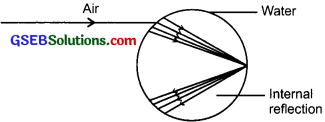Question 12.
A short-sighted person cannot see clearly beyond 5 m. Calculate the power of the lens required to correct his vision to normal?
$$\frac {1}{v}$$ – $$\frac {1}{u}$$ = $$\frac {1}{f}$$
$$\frac {1}{-5}$$ – $$\frac {1}{-∞}$$ = $$\frac {1}{ 3}$$
f = – 5m
p = $$\frac {1}{f}$$ = $$\frac {1}{-5}$$ = -0.20
power = -0.2 DioptreQuestion 13.
Why can’t we see the object very close to our eye?
The objects are seen only when the image forms on the retina when the light rays pass through the lens. The lens has its fixed ability to change the focal length with the help of ciliary muscles. Ciliary muscles cannot be contracted beyond a certain limit to change the focal length of the eye lens. The objects kept very close to our eye cannot be focused by ciliary muscles.

Question 14.
What is night blindness and colour blindness?
When a person lacks rod cells in retina he is not able to see the objects clearly in less/dim light, such a defect is called night blindness. When a person lacks cone cells in retina he is not able to see/distinguish between different colours, such a defect of eye is called as colour blindness.

Question 15.
What is myopia? How can it be corrected?
Myopia is an eye defect also called short-sightedness. In this type of defect, a person can see nearby objects clearly but cannot see far off objects clearly. It may be caused due to the increase in the size of the eyeball or due to the decrease in the focal length of the eye lens. Correction It can be corrected by using the concave lens of appropriate focal length.

Question 16.
What is hypermetropia? How can it be corrected?
Hypermetropia is an eye defect also called as long-sightedness. A person can see a far off objects but cannot see nearby objects. It is because the image is formed beyond the retina.
Cause –
(a) The focal length of the eye lens is too long.
(b) The eyeball becomes too small.
Correction: It can be corrected by using a convex lens of appropriate power.Question 17.
What is presbyopia? How can it be corrected?
Presbyopia is caused due to a decrease in the power of accommodation of the eyes due to ageing and weakening of ciliary muscles, diminishing flexibility of eye lens. A person with this defect cannot see nearby as well as far off objects clearly.
Correction: k can be corrected by using a bi-focal lens with both concave and convex lens in it.

Question 18.
Give the difference between myopia and hypermetropia.
Myopia:

• Short-sightedness – can see the nearby object but cannot see far off objects.
• Image is formed in front of the retina.
• The size of the eyeball increases.
• The focal length of the eye lens decreases.
• Corrected by using a concave lens.

Hypermetropia:

• Long-sightedness – can see far off objects but cannot see nearby objects.
• Image is formed beyond the retina.
• The size of the eyeball decreases.
• The focal length of the eye lens increases.
• Corrected by using a convex lens.

Question 19.
Distinguish between presbyopia and hypermetropia.
hypermetropia:

• Only far-sightedness.
• Eyeball becomes short or the focal
• length of the eye lens increases.
• Corrected by using a convex lens.

Presbyopia:

• Both far and short-sightedness.
• Ciliary muscles become weak and unable to
• Corrected by using a bifocal lens.Question 20.
The near point of a hypermetropic eye is 80 cm. What is the nature and power of the lens required to enable him to read a book placed at 25 cm from the eyes?
Near point = -80 cm
Object distance u = – 25 cm
v = -80 cm (convex lens in case of hypermetropia)
$$\frac {1}{f}$$ = $$\frac {1}{v}$$ – $$\frac {1}{u}$$ = $$\frac {1}{(-80)}$$ – $$\frac {1}{(-25)}$$
$$\frac {1}{f}$$ = $$\frac {-1}{80}$$ + $$\frac {1}{25}$$ = $$\frac {-5 + 16}{400}$$
$$\frac {1}{f}$$ = $$\frac {+11}{400}$$
f = $$\frac {400}{11}$$ = 36.36 cm
f = 0.36 m
power of lens p = $$\frac {1}{f}$$ = $$\frac {1}{ 0.36}$$ = 2.78 D

Question 21.
What is meant by the dispersion of white light? Draw a ray diagram to show the dispersion of white light by a glass prism. Give a reason why do we get different colours of light?
Dispersion of light: The splitting of white light into seven colours on passing through a transparent medium like glass prism is called dispersion of light.

Diagram: Dispersion of light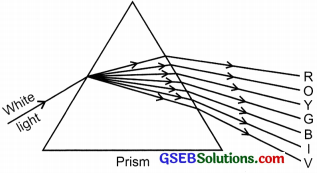We get different colours because each colour of light has different bending ability when they pass through the glass prism due to their different speed.Question 22.
A student can see objects clearly only when the objects are lying at distances between 60 cm and 320 cm from the eye.
(a) What kind of eye defect he is suffering from?
(b) What kind of lens will be required to increase his range from 25 cm to infinity? Explain briefly.
(a) The student is suffering from myopia and hypermetropia both.
(b) It can be corrected by using the bifocal lens of appropriate focal length and power.

Question 23.
When we see any object through the hot air over the fire, it appears to be wavy moving slightly. Explain.
The objects beyond the hot air appear to be wavy because the medium for light to pass through changes, the light passes from denser to rarer and then again to denser medium thereby causing refraction in the air. Moreover, the refractive index of the hot air keeps changing which leads to giving the wavy appearance of the object.

Question 24.
Study the diagram given below and answer the questions that it follows:
(a) Name the defect and give the reason.
(b) Give 2 causes for this defect.
(c) Give the correction – draw a diagram for the same.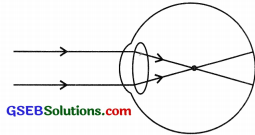(a) The defect is myopia or short-sightedness as the image formed in front of the retina.
(b) It is caused due to the decrease in the focal length of the eye lens and an increase in the size of the eyeball.
(c) The defect can be corrected by Virtual image formed at F using the concave lens.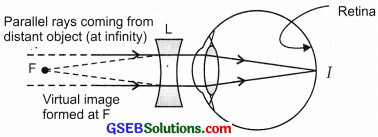Question 25.
In the given diagram label A, B, C and D and give the function of B and D.
A = Cornea
B = Ciliary muscles A
C = Retina
D = Optic nerve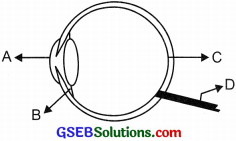The function of B and D are:
B : Ciliary muscles: It helps in holding the eye lens and changing or adjusting the focal length of the lens.
D : Optic nerve: It sends the electrical signal from retina to the brain.

Question 26.
Draw a labelled diagram of rainbow formation. Also, explain the phenomenon of rainbow formation.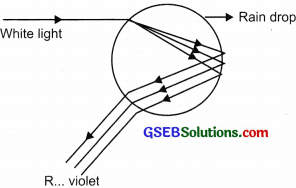Water droplets act as a tiny prism in the SK The sunlight when enters these tiny droplets undergo internal reflection and also refract these rays which are dispersed causing a band of seven colours called rainbow. Rainbow is always formed in the
direction opposite to the sun.Question 27.
What do understand by the term pupil reflex? Explain with the diagram.
The changes in the size of the pupil to control the amount of light entering the eye is called pupil reflex. In bright light, pupil size is reduced as too much light falling on the retina could damage it. The reduced size of the pupil allows only a limited amount of light to enter.  In the dim light, pupil size is increased to allow as much light as possible to enter the eye.

The retina detects the brightness of light that is entering the eye. An impulse pass to the brain along sensory neurons and the message travels back to the muscles of the iris by motor neurons, triggering a response – the change in the size of the pupil due to contraction of radial or circular muscles.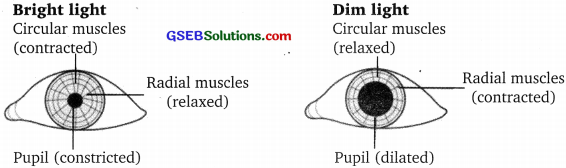Question 28.
What is the difference in the circular muscles and the ciliary muscles in the human eye?
The circular muscle controls the size of the iris and the ciliary muscles control the size of the eye lens.Question 29.
A person needs a lens of power 5.5 D for correcting his distant vision. For correcting his near vision he needs a lens of power + 1.5 D. What is the focal length of the lens required for correcting (i) distant vision, and (ii) near vision?
(i) Power of lens needed for correction distant vision of the person P2 = 5.5 D
Focal length of lens required for correcting distant vision –
f1 = i/p1 = 1/- 5.5 = -18 cm

(ii) For correcting near vision power required P2 = + 1.5 D
Focal length of lens required for correcting near vision
f1 = I/P2 = 1/-1.5 = 0.666 m = 66.7 cm

Question 30.
The far point of a myopic person is 80 cm in front of the eye. What is the nature and power of the lens required to correct the problem?
To correct myopia the person concerned should use a concave lens (diverging lens) of suitable focal length.
For myopic eye: Far point of normal eye = u = at infinity = -∞
The virtual image is formed at the far point of myopic person
v = – 80 cm From lens formula,
$$\frac {1}{f}$$ = $$\frac {1}{v}$$ – $$\frac {1}{u}$$
$$\frac {1}{f}$$ = $$\frac {1}{-80}$$ – $$\frac {1}{-∞}$$
f = – 80 cm , – 0.80 m
Power of the lens P = $$\frac {1}{f}$$ = $$\frac {-100}{80}$$ = – 1.25 DQuestion 31.
Describe and explain the action of eye changes seen while changing the focus from a distant object to a near object.
The ciliary muscle needs to contracts to see the nearby objects and focus the image on the retina correctly. When the eye is focusing on the distant object the ciliary muscles are relaxed. But to focus the nearby object the ciliary muscle contract and the suspensory ligaments become relaxed. The eye lens changes its focal length and becomes more convex in nature to obtain the image on the retina.

Question 32.
State the difference between rods and cones cells in the retina of the human eye, Draw a neat diagram of the human eye.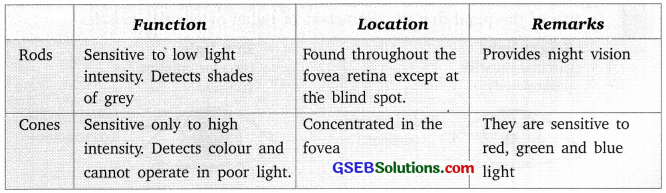Fovea: In the eye, a tiny pit located in the macula of the retina that provides the clearest vision of all. Only in the fovea are the layers of the retina spread aside to let the light fall directly on the cones, the cells that give the sharpest image.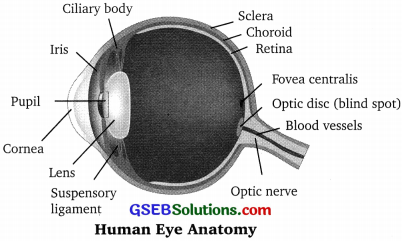Question 1.
Draw a neat labelled diagram of the human eye and explain the working of each part of it.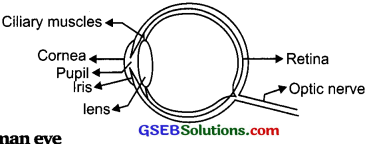Working of Human eye
The human eye consists of various parts which help us in seeing the objects, the function of various parts are:
(a) Cornea: Ir is the transparent membrane which refracts the light entering our eye.
(b) Iris: Iris controls the size of the pupil.
(c) Pupil: It allows the light entering our eye to pass through it.
(d) Lens: Refracts the light of the objects at different places on the retina.
(e) Ciliary muscles: Helps in changing the focal length of the lens.
(f) Retina: It is the screen of the eye on which image is formed. It consists of rods and cones.
(g) Optic nerve: k carries the electrical signals from the retina to the brain.Question 2.
Describe with the help of a diagram, how the refraction of light takes place through a glass prism.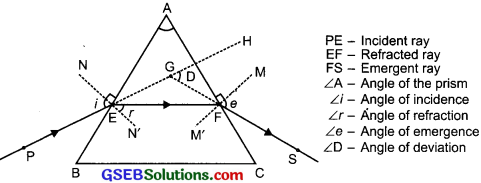The incident ray PE when enters the prism it gets refracted, bends and forms ∠r wit the normal. The angle of refraction is smaller than the angle of incidence. The incident ray bends towards the normal, as it passes from rarer medium to denser medium. When this refracted ray passes from denser medium to rarer medium it bends away from the normal. This emergent ray has bent out at an angle to the direction of the incident ray. This angle is called the angle of deviation ∠D.

Question 3.
Name three refractive defects of vision with the help of a diagram. Explain the reasons for and correction of these defects.
The three refractive defects of vision are –

1. Myopia
2. Hypermetropia
3. Presbyopia

1. Myopia:
(a) Myopia or short-sightedness – Eye can see objects at a short distance. The inability of the eye in viewing long distant objects. The image falls before the retina.

Causes:
1. Elongation of the eyeball.
2. Excessive curvature in cornea and focal length of lens decreases.

Correction:
By using a concave lens (diverging lens), which shifts the image to the retina by diverging the rays further.
(b) Myopic eye, the image is formed in front of the retina.

2.  Hypermetropia:
Also called long-sightedness. A person can see long distant objects but cannot see nearby objects distinctly. This is because the eyeball becomes smaller, focal length increases. It can be corrected by using a converging lens called a convex lens.

3. Presbyopia:
Presbyopia: The person suffering from this disease cannot see nearby as well as far off objects. It is caused due to the weakening of ciliary muscles. This defect is caused due to ageing. The lens is not able to change the focal length. It can be corrected by using the bi-focal lens.

Questions On High Order Thinking Skills (Hots)

Question 1.
How does the size of particles present in the medium produce different colours of light by its scattering property?
The colour of the scattering light in a medium depends on the size of the scattering particles.

• If the size of the particles is very small, it will scatter mainly blue light.
• If the size of particles is larger then it will scatter light of longer wavelength i.e., red.
• If the size of scattering particles is larger enough, the light appears to be white.Question 2.
Give one use of the following properties of light:

• Scattering of light
• Persistence of vision
• Power of accommodation
• Refraction of light
• Reflection of light

• Due to the scattering of light, we can see the different colours of the sky and rainbow formation.
• Used in cinematography.
• The eye can see both nearby and far off objects from the same point.
• We can see through lenses, eye defects can be corrected, we can see underwater.
• Our eyes can see an object only due to reflection of light by the object into our eyes.

Question 3.
To correct myopia why we use the concave lens and to correct hypermetropia, why do we use convex lens? Why can’t we do vice-versa?